Ex 11.2

Chapter 11 Class 10 Constructions [Deleted]
Serial order wise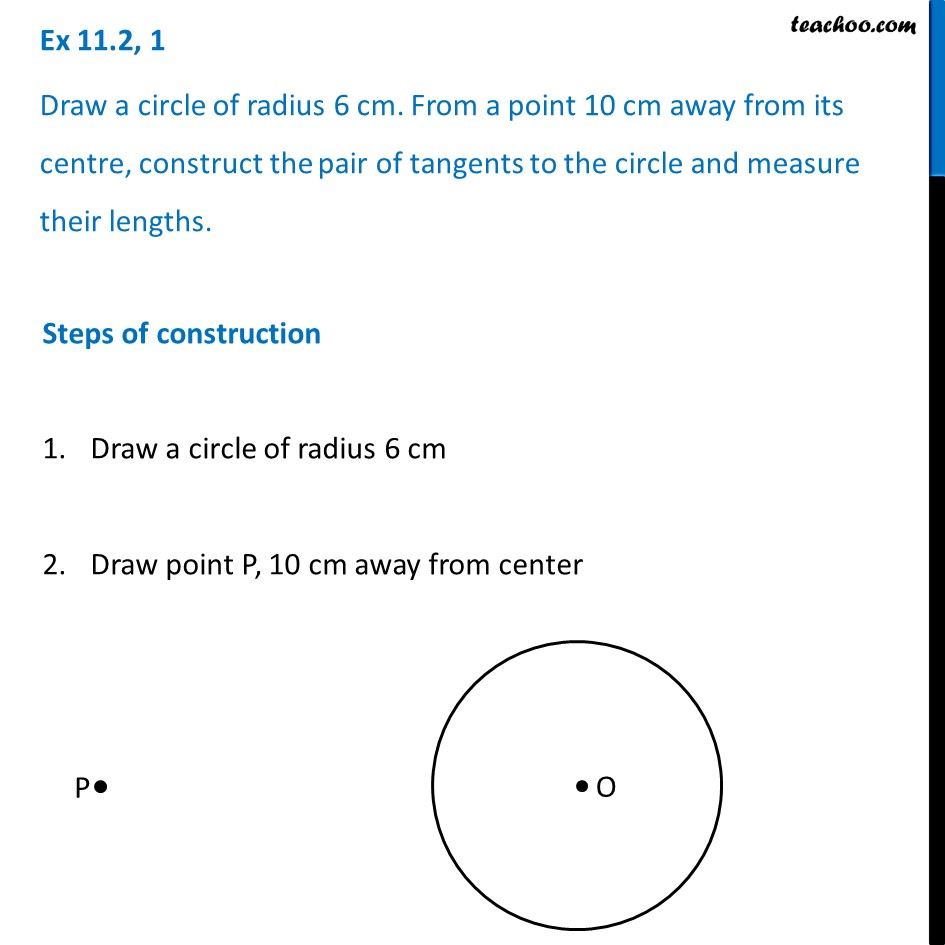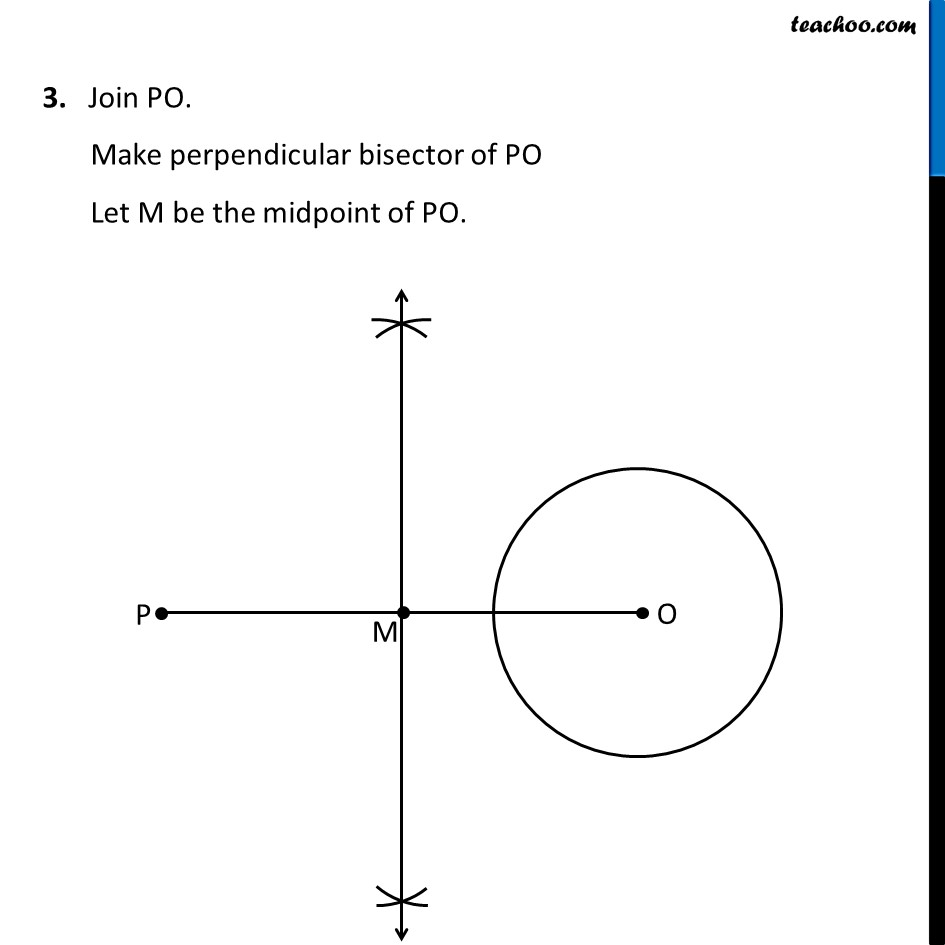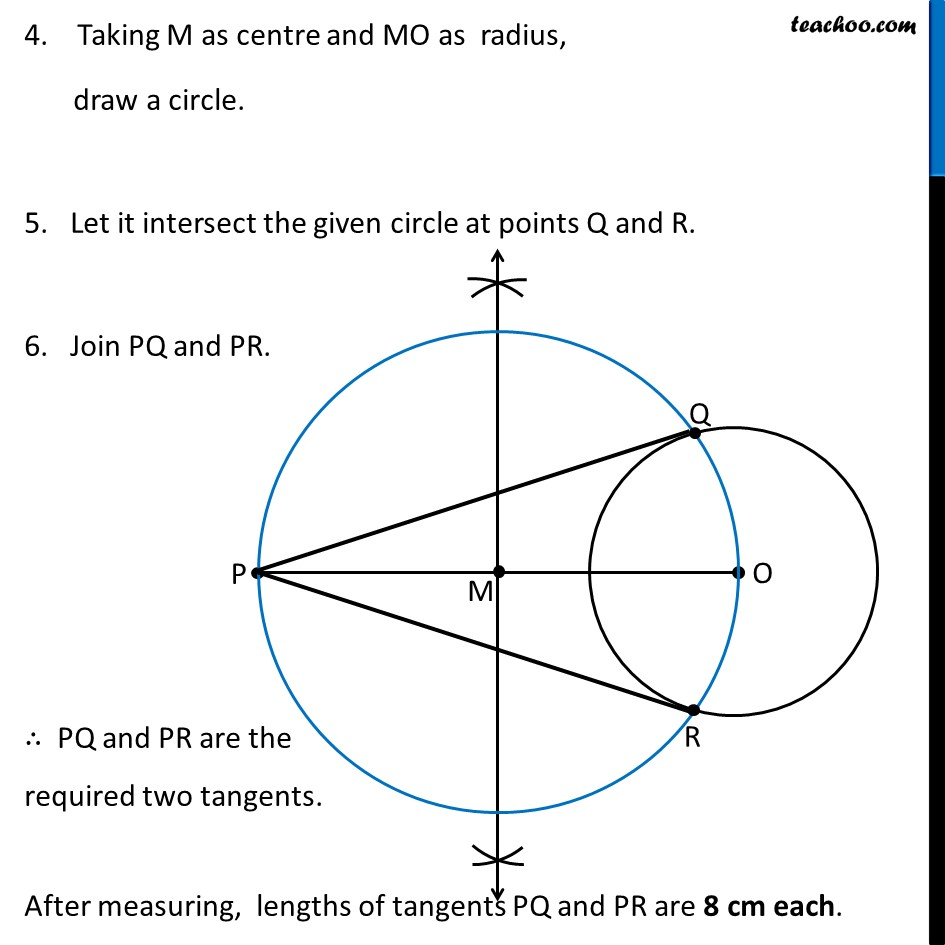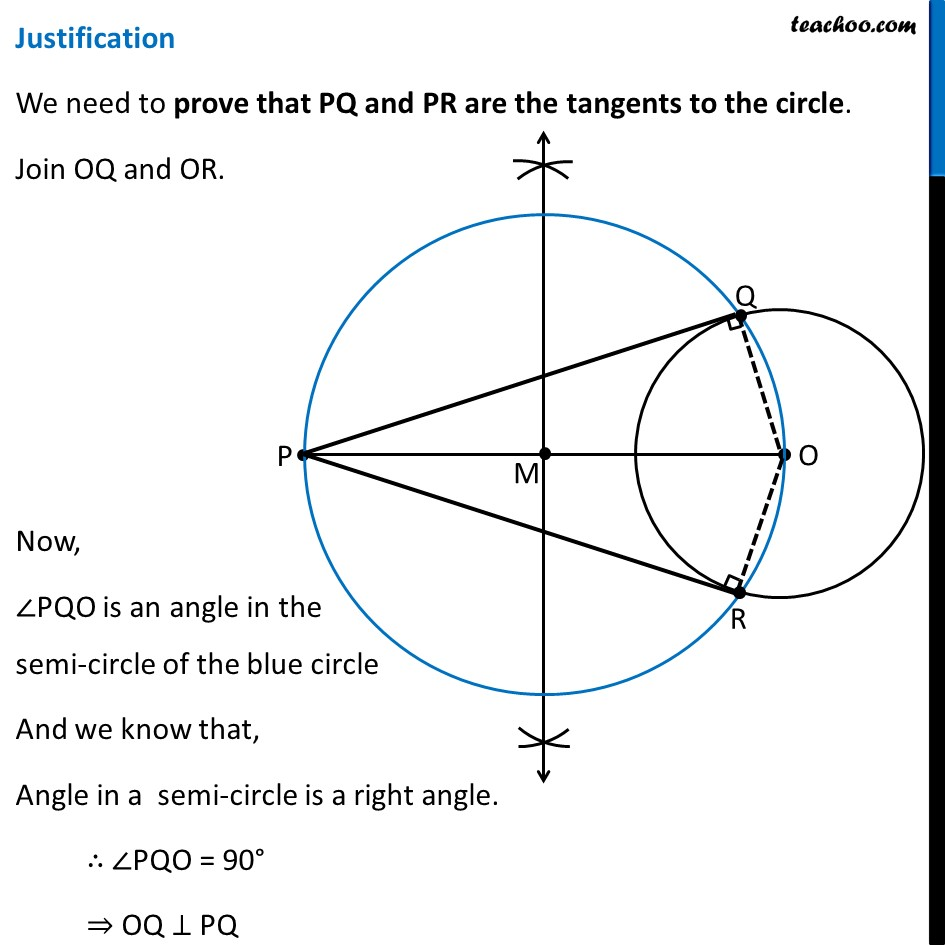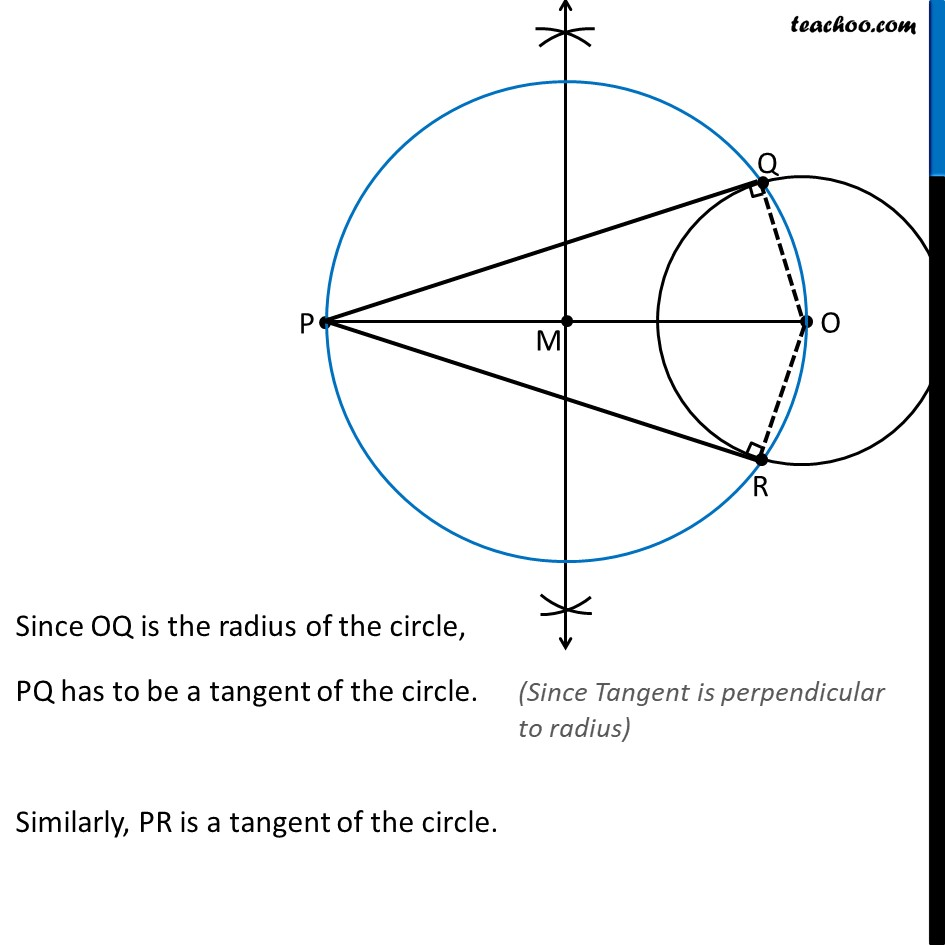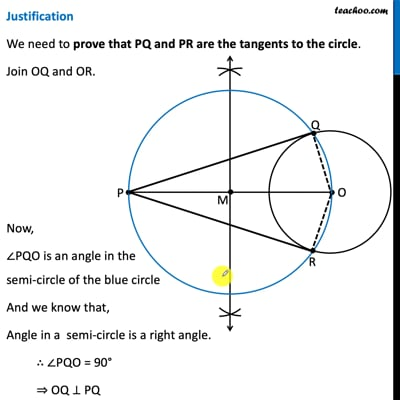This video is only available for Teachoo black users

Maths Crash Course - Live lectures + all videos + Real time Doubt solving!

### Transcript

Ex 11.2, 1 Draw a circle of radius 6 cm. From a point 10 cm away from its centre, construct the pair of tangents to the circle and measure their lengths. Steps of construction Draw a circle of radius 6 cm Draw point P, 10 cm away from center 3. Join PO. Make perpendicular bisector of PO Let M be the midpoint of PO. 4. Taking M as centre and MO as radius, draw a circle. 5. Let it intersect the given circle at points Q and R. 6. Join PQ and PR. ∴ PQ and PR are the required two tangents. After measuring, lengths of tangents PQ and PR are 8 cm each. 4. Taking M as centre and MO as radius, draw a circle. 5. Let it intersect the given circle at points Q and R. 6. Join PQ and PR. ∴ PQ and PR are the required two tangents. After measuring, lengths of tangents PQ and PR are 8 cm each. Justification We need to prove that PQ and PR are the tangents to the circle. Join OQ and OR. Now, ∠PQO is an angle in the semi-circle of the blue circle And we know that, Angle in a semi-circle is a right angle. ∴ ∠PQO = 90° ⇒ OQ ⊥ PQ Since OQ is the radius of the circle, PQ has to be a tangent of the circle. Similarly, PR is a tangent of the circle